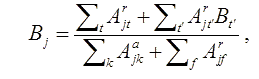### Dielectronic Recombination (DR) Rate Coefficient

The total DR rate coefficient averaged over a Maxwell-Boltzmann electron energy distribution at temperature T is given bywhere gi and gj are the statistical weights of the states i and j, Eij is the resonance energy, Ajia is the autoionization rate from the states j to i, Ry is the Rydberg energy, kB is the Boltzman constant, and a0 is the Bohr radius. Bj denotes the radiative stabilizing branching ratio and can be expressed aswhere the final states t and t' are below and above the ionization threshold, respectively. Bt' is the branching ratio for radiative stabilization of t and can be determined by evaluation Bj iteratively.
For convenience in plasma modeling we have fitted our total DR rate coefficients by the formulawhere T is in units of eV.